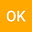NEWTON'S FORMULA AND A DIFFERENT VERSION OF LAGRANGE INTERPOLATION FORMULA
•• osman kucuk
osman kucuk
Harmony Public Schools

Corresponding Author:[email protected]

Author Profile## Abstract

Finding the nth term of a sequence is one of the most common questions in Algebra. This article introduces an original and alternative formula to calculate any term of any degree sequence. The examples are solved by using this formula first, then the answers are confirmed by using either Newton's Formula or Lagrange Interpolation Formula. Both the formula and its proof take advantage of Pascal's triangle.